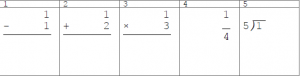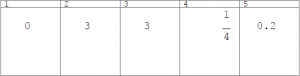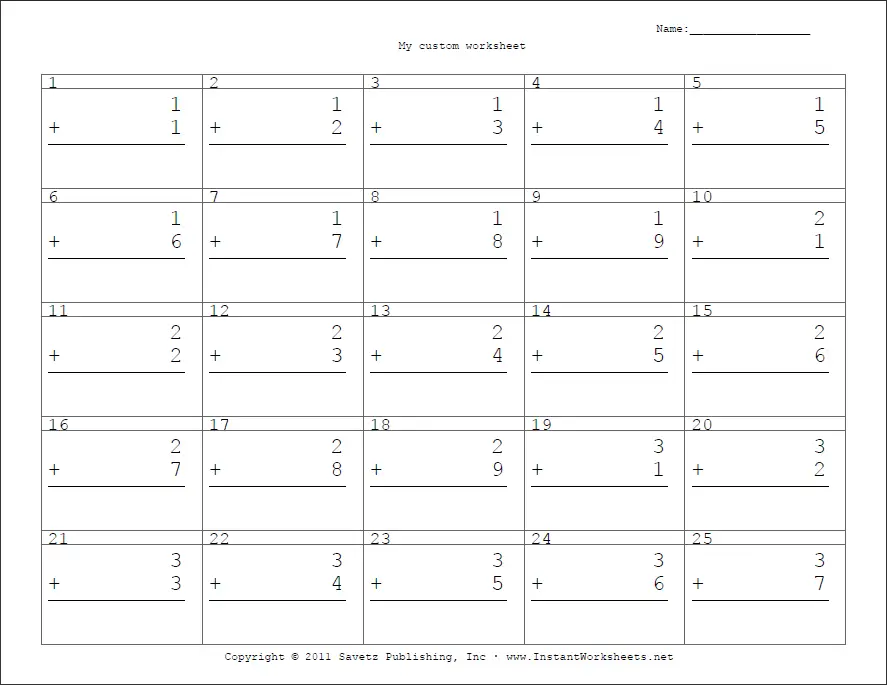# Non-fraction arithmetic and simplify fraction worksheet

This generator creates custom non-fraction arithmetic and simplify fraction worksheetsQuestion sample imageSample image - landscape

Operation/Question Type: Select the type of questions to appear on the worksheet.

• Perfect division: When used in place of division, it will only include division questions that are perfectly divisible. When used in combination with the “Round to digit” parameter, it will also include questions that are perfectly divisible up to the digit specified.
• Simplify: will arrange number in the form of a fraction. The simplified equivalent of the fraction will appear on the answer sheet

Operation Options:

• Single Operation: Only the first operation selected will be used.
• Random Operations: Will randomly use selected operations
• Evenly mixed: Will try to ensure an even number of questions per operation(with the exception of perfect division)

First and Second Number: Set the range of the first and second number. First number is the number on the left side of the operation, and second number on the right side. In the case of simplify, first number will be on top, and second number on the bottom.

Round to digit: The answers will be rounded to the specified digit.

Increment: This represents the size of the step between questions. For example if your first number has the minimum of 1, and maximum of 6. With an increment of 1, the numbers 1, 2, 3, 4, 5, and 6 would be used as first numbers; with an increment of 2, the numbers 1, 3, 5 would be used; with an increment of 3, the numbers 1 and 4 would be used.

Question progression:

• Sequential: will result in the numbers from min to max to be used in ascending order.
• Random: will randomize the order appearance of the numbers.